StatLect

# Continuous Mapping theorem

The Continuous Mapping theorem states that stochastic convergence is preserved by continuous functions.## The problem

Suppose that a sequence of random vectorsconverges to a random vector(in probability, in distribution or almost surely).

Now, take a transformed sequence, whereis a function.

Under what conditions isalso a convergent sequence?

The Continuous Mapping theorem states that stochastic convergence is preserved ifis a continuous function.

## The theorem

Here is a statement of the multivariate version of the Continuous Mapping theorem.

Proposition Letbe a sequence of-dimensional random vectors. Letbe a continuous function. Then,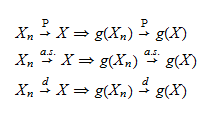wheredenotes convergence in probability,denotes almost sure convergence anddenotes convergence in distribution.

Proof

See, e.g., Shao (2003).

## Consequences

The next sections present some important consequences of the Continuous Mapping theorem.

### Sums and products of sequences converging in probability

An important implication of the Continuous Mapping theorem is that arithmetic operations preserve convergence in probability.

Proposition Ifand. Then,Proof

First of all, note that convergence in probability ofand ofimplies their joint convergence in probability (see the lecture entitled Convergence in probability), that is, their convergence as a vector: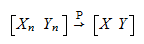Now, the sum and the product are continuous functions of the operands. Thus, for example,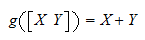is a continuous function, and, by using the Continuous Mapping theorem, we obtainwheredenotes a limit in probability.

### Sums and products of sequences converging almost surely

Everything that was said in the previous subsection applies, with obvious modifications, also to almost surely convergent sequences.

Proposition Ifand, thenProof

Similar to previous proof. Just replace convergence in probability with almost sure convergence.

### Sums and products of sequences converging in distribution

For convergence almost surely and convergence in probability, the convergence ofandindividually implies their joint convergence as a vector (see the previous two proofs), but this is not the case for convergence in distribution. Therefore, to obtain preservation of convergence in distribution under arithmetic operations, we need the stronger assumption of joint convergence in distribution.

Proposition If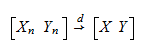then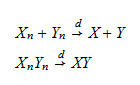Proof

Again, similar to the proof for convergence in probability, but this time joint convergence is already in the assumptions.

## More details

The following sections contain more details about the Continuous Mapping theorem.

### Convergence of ratios

As a byproduct of the propositions stated above, we also have the following proposition.

Proposition If a sequence of random variablesconverges to, thenprovidedis almost surely different from(we did not specify the kind of convergence, which can be in probability, almost surely or in distribution).

Proof

This is a consequence of the Continuous Mapping theorem and of the fact thatis a continuous function for.

An immediate consequence of the previous proposition follows.

Proposition If two sequences of random variablesandconverge toandrespectively, thenprovidedis almost surely different from. Convergence can be in probability, almost surely or in distribution (but the latter requires joint convergence in distribution ofand).

Proof

This is a consequence of the fact that the ratio can be written as a product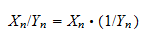The first operand of the product converges by assumption. The second converges because of the previous proposition. Therefore, their product converges because convergence is preserved under products.

### Random matrices

The Continuous Mapping theorem applies also to random matrices because random matrices are just random vectors whose entries have been arranged into the columns of a matrix.

In particular:

• if two sequences of random matrices are convergent, then also the sum and the product of their terms are convergent (provided their dimensions are such that they can be summed or multiplied);

• if a sequence of square random matricesconverges to a random matrix, then the sequence of inverse matricesconverges to the random matrix(provided the matrices are invertible). This is a consequence of the fact that matrix inversion is a continuous transformation.

## Applications

The Continuous Mapping theorem has several important applications. For example, it is used to prove:

## Solved exercises

Below you can find some exercises with explained solutions.

### Exercise 1

Consider a sequenceof random variables converging in distribution to a random variablehaving a standard normal distribution.

Consider the functionwhich is a continuous function.

Find the limit in distribution of the sequence.

Solution

The sequenceconverges in distribution toby the Continuous Mapping theorem. But the square of a standard normal random variable has a Chi-square distribution with one degree of freedom. Therefore, the sequenceconverges in distribution to a Chi-square distribution with one degree of freedom.

## References

Shao, J. (2007) Mathematical statistics, Springer.